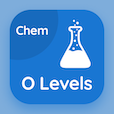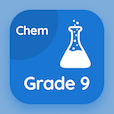Cambridge Online Courses (GCSE)

O Level Chemistry Quizzes

O Level Chemistry Quiz - Complete

Practice Electrolyte and Non Electrolyte quiz questions and answers, electrolyte and non electrolyte MCQ questions PDF, test 6 to study O Level Chemistry online course. Electricity and Chemistry MCQ questions, electrolyte and non electrolyte Multiple Choice Questions (MCQ Quiz) for online college degrees. Electrolyte and Non Electrolyte Book PDF: chemical symbols, chemical reactions, ionic compounds: crystal lattices, measuring speed of reaction, electrolyte and non electrolyte test prep for SAT subject test tutoring.

"Reactivity series of metals is also called as the" Quiz PDF: electrolyte and non electrolyte App APK with electrochemical series, preferential series, electrolytic series, and selective discharge series choices for ACT prep classes. Solve electricity and chemistry questions and answers to improve problem solving skills for online degrees.

## Trivia Questions on Electrolyte & Non Electrolyte MCQs

MCQ: Reactivity series of metals is also called as the

preferential series
electrochemical series
electrolytic series
selective discharge series

MCQ: Rate of reaction can be calculated by dividing the change in mass or volume by

time taken
total mass
amount of volume
the temperature

MCQ: In the formation of an ionic bond in Potassium Fluoride (KF), the Potassium ion (K+) gets a

single positive charge
double positive charge
single negative charge
double negative charge

MCQ: NaOH + HCl →

Na(OH)2 + H2
NaCl + H2
NaCl + H2O
NaCl + H2 + O2

MCQ: Valency of carbonate ion CO3 is

-1
-2
-3
-4

### More Quizzes from O Level Chemistry Book